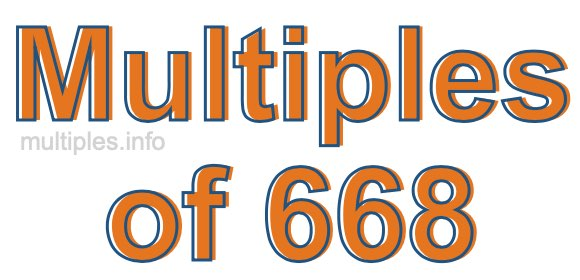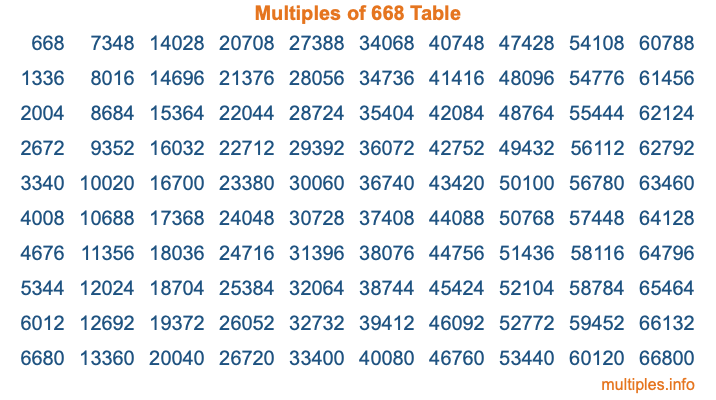Multiples of 668Welcome to the Multiples of 668 page. Here we will first teach you everything you will ever need to know about the multiples of 668, and then give you a study guide summary of everything we taught you to make sure you remember it all. Use this page to look up facts and learn information about the multiples of 668. This page will make you a multiples of six hundred sixty-eight expert!

Definition of Multiples of 668
Multiples of 668 are all the numbers that when divided by 668 equal an integer. Each of the multiples of 668 are called a multiple. A multiple of 668 is created by multiplying 668 by an integer.

Therefore, to create a list of multiples of 668, you start with 1 multiplied by 668, then 2 multiplied by 668, then 3 multiplied by 668, and so on for as long as you want. Thus, the list of the first five multiples of 668 is 668, 1336, 2004, 2672, and 3340. To see a larger list of multiples of 668, see the printable image of Multiples of 668 further down on this page. We also have a category where you can choose any nth multiple of 668.

Multiples of 668 Checker
The Multiples of 668 Checker below checks to see if any number of your choice is a multiple of 668. In other words, it checks to see if there is any number (integer) that when multiplied by 668 will equal your number. To do that, we divide your number by 668. If the the quotient is an integer, then your number is a multiple of 668.

Is  a multiple of 668?

Least Common Multiple of 668 and ...
A Least Common Multiple (LCM) is the lowest multiple that two or more numbers have in common. This is also called the smallest common multiple or lowest common multiple and is useful to know when you are adding our subtracting fractions. Enter one or more numbers below (668 is already entered) to find the LCM.

Check out our LCM Calculator if you need more details about the Least Common Multiple or if you need the LCM for different numbers for adding and subtraction fractions.

nth Multiple of 668
As we stated above, 668 is the first multiple of 668, 1336 is the second multiple of 668, 2004 is the third multiple of 668, and so on. Enter a number below to find the nth multiple of 668.

th multiple of 668

Multiples of 668 vs Factors of 668
668 is a multiple of 668 and a factor of 668, but that is where the similarities end. All postive multiples of 668 are 668 or greater than 668. All positive factors of 668 are 668 or less than 668.

Below is the beginning list of multiples of 668 and the factors of 668 so you can compare:

Multiples of 668: 668, 1336, 2004, 2672, 3340, etc.

Factors of 668: 1, 2, 4, 167, 334, 668

As you can see, the multiples of 668 are all the numbers that you can divide by 668 to get a whole number. The factors of 668, on the other hand, are all the whole numbers that you can multiply by another whole number to get 668.

It's also interesting to note that if a number (x) is a factor of 668, then 668 will also be a multiple of that number (x).

Multiples of 668 vs Divisors of 668
The divisors of 668 are all the integers that 668 can be divided by evenly. Below is a list of the divisors of 668.

Divisors of 668: 1, 2, 4, 167, 334, 668

The interesting thing to note here is that if you take any multiple of 668 and divide it by a divisor of 668, you will see that the quotient is an integer.

Multiples of 668 Table
Below is an image of the first 100 multiples of 668 in a table. The table is in chronological order, column by column. The first column has the first ten multiples of 668, the second column has the next ten multiples of 668, and so on.The Multiples of 668 Table is also referred to as the 668 Times Table or Times Table of 668. You are welcome to print out our table for your studies.

Negative Multiples of 668
Although not often discussed or needed in math, it is worth mentioning that you can make a list of negative multiples of 668 by multiplying 668 by -1, then by -2, then by -3, and so on, to get the following list of negative multiples of 668:

-668, -1336, -2004, -2672, -3340, etc.

Multiples of 668 Summary
Below is a summary of important Multiples of 668 facts that we have discussed on this page. To retain the knowledge on this page, we recommend that you read through the summary and explain to yourself or a study partner why they hold true.

There are an infinite number of multiples of 668.

A multiple of 668 divided by 668 will equal a whole number.

668 divided by a factor of 668 equals a divisor of 668.

The nth multiple of 668 is n times 668.

The largest factor of 668 is equal to the first positive multiple of 668.

668 is a multiple of every factor of 668.

668 is a multiple of 668.

A multiple of 668 divided by a divisor of 668 equals an integer.

668 divided by a divisor of 668 equals a factor of 668.

Any integer times 668 will equal a multiple of 668.

Multiples of a Number
Here you can get the multiples of another number, all with the same attention to detail as we did for multiples of 668 on this page.

Multiples of
Multiples of 669
Did you find our page about multiples of six hundred sixty-eight educational? Do you want more knowledge? Check out the multiples of the next number on our list!